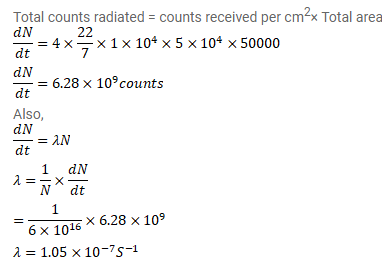# A point source emitting alpha particles is placed at a distance of

Question:

A point source emitting alpha particles is placed at a distance of $1 \mathrm{~m}$ from a counter which records any alpha particle falling on its $1 \mathrm{~cm}^{2}$ window. If the source contains $6.0 \times 10^{16}$ active nuclei and the counter records a rate of 50000 counts/second, find the decay constant. Assume that the source emits alpha particles uniformly in all directions and the alpha particles fall nearly normally on the window.

Solution: